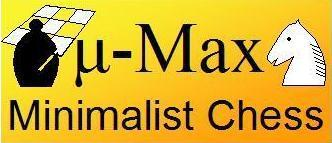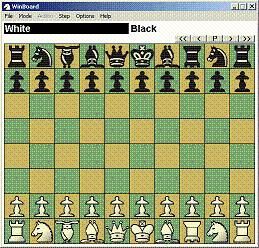## Micro-Max, a 133-line Chess Source```Previous   Next   Version 4   Downloads   Fairy-Max
```## Newly released beta version!

There now is a Winboard version that can handle bigger boards, and a Fairy-Max engine that can run under it (download)

The GUI knows the rules of Capablanca Chess, while Fairy-Max can play a wide variety of board sizes and piece types, as it allows user-defined pieces.

My original aim was to write a chess program smaller than 1024 characters. I could not do it, so far. Even when I dropped the nitty gritty details of the FIDE rules, like castling and en-passant capture, I could not get the size much below 1200 characters.

So I shifted my aim somewhat, and wrote something less minimalistic in up to 2000 characters of source-code. This gave me enough space to implement a (hash) transposition table, checking of the legality of the input moves, and full FIDE rules. Except for under-promotions, which I considered a dull input problem, since the program is unlikely to ever find itself in a situation where it would be useful to play one.

(For real purists: a close-to-minimal version that does understand full FIDE rules including under-promotion can be found here. It measures 1433 characters. The under-promotions are implemented in a single line that wastes 32 characters. To play one, type 1, 2, or 3 as the 5th character of the input move, for promotion to R, B, or N, respectively. If you type nothing, or 0, promotion is to Q.)

As far as I am aware, this still makes micro-Max the smallest C Chess program in existence. A close competitor for this honor, Toledo, measures 2168 characters. Despite its smaller size, micro-Max seems to beat Toledo easily.

On these pages various aspects of micro-Max are described:

 Basic Data Representations Move Generation Search Algorithm Transposition Table Evaluation The Interface
An overview of the meaning of all variables in the program can be found here. To make it easier for those who want to study the algorithm, there now also is a version that uses more meaningful (and much longer...) variable names.

If you want to try micro-Max on your PC, you can copy-paste the source and compile it yourself. Details on how to do this can be found here.

#### Future

There are still plenty places where I can scavenge a few characters off the source code. (E.g. `A->K` in stead of `A.K`, and `a->X&M^M` in stead of `(a->X&M)!=M`, and perhaps combining the two Zobrist keys in a 64-bit type.) The castling code is also rather dumb and bulky. I hope to be able to compact the code enough (without loss of functionality) to make room for new features, in particular null-move threat detection. I will post the progress of this project regularly on separate pages, so that it does not mess up the tutorial on micro-Max 3.2. If some clearly defined feature is added to future versions of micro-Max, the page explaining it will be included in the index above.

Below is the complete source code of micro-Max 3.2. (Click on the various code lines to go directly to their explanation.) If you want to copy-paste it, it is recommended you do it from here, because if I correct a small bug or typo I am generally too lazy to do it on all other pages where the source occurs. So I do it here, and on the page where the particular feature needing the correction is discussed and highlighted.

```/***************************************************************************/
/*                               micro-Max,                                */
/* A chess program smaller than 2KB (of non-blank source), by H.G. Muller  */
/***************************************************************************/
/* version 3.2 (2000 characters) features:                                 */
/* - recursive negamax search                                              */
/* - quiescence search with recaptures                                     */
/* - recapture extensions                                                  */
/* - (internal) iterative deepening                                        */
/* - best-move-first 'sorting'                                             */
/* - a hash table storing score and best move                              */
/* - full FIDE rules (expt minor ptomotion) and move-legality checking     */

#define F(I,S,N) for(I=S;I<N;I++)
#define W(A) while(A)
#define K(A,B) *(int*)(T+A+(B&8)+S*(B&7))
#define J(A) K(y+A,b[y])-K(x+A,u)-K(H+A,t)

#define U 16777224
struct _ {int K,V;char X,Y,D;} A[U];           /* hash table, 16M+8 entries*/

int V=112,M=136,S=128,I=8e4,C=799,Q,N,i;       /* V=0x70=rank mask, M=0x88 */

char O,K,L,
w[]={0,1,1,3,-1,3,5,9},                        /* relative piece values    */
o[]={-16,-15,-17,0,1,16,0,1,16,15,17,0,14,18,31,33,0, /* step-vector lists */
7,-1,11,6,8,3,6,                          /* 1st dir. in o[] per piece*/
6,3,5,7,4,5,3,6},                         /* initial piece setup      */
b,                                        /* board: half of 16x8+dummy*/
T,                                       /* hash translation table   */

n[]=".?+nkbrq?*?NKBRQ";                        /* piece symbols on printout*/

D(k,q,l,e,J,Z,E,z,n)    /* recursive minimax search, k=moving side, n=depth*/
int k,q,l,e,J,Z,E,z,n;  /* (q,l)=window, e=current eval. score, E=e.p. sqr.*/
{                       /* e=score, z=prev.dest; J,Z=hashkeys; return score*/
int j,r,m,v,d,h,i=9,F,G;
char t,p,u,x,y,X,Y,H,B;
struct _*a=A;
/* lookup pos. in hash table*/
j=(k*E^J)&U-9;                                /* try 8 consec. locations  */
W((h=A[++j].K)&&h-Z&&--i);                    /* first empty or match     */
a+=i?j:0;                                     /* dummy A if miss & full*/
if(a->K)                                      /* hit: pos. is in hash tab */
{d=a->D;v=a->V;X=a->X;                        /* examine stored data      */
if(d>=n)                                     /* if depth sufficient:     */
{if(v>=l|X&S&&v<=q|X&8)return v;             /* use if window compatible */
d=n-1;                                      /* or use as iter. start    */
}X&=~M;Y=a->Y;                               /*      with best-move hint */
Y=d?Y:0;                                     /* don't try best at d=0    */
}else d=X=Y=0;                                /* start iter., no best yet */
N++;                                          /* node count (for timing)  */
W(d++<n|z==8&N<1e7&d<98)                      /* iterative deepening loop */
{x=B=X;                                       /* start scan at prev. best */
Y|=8&Y>>4;                                   /* request try noncastl. 1st*/
m=d>1?-I:e;                                  /* unconsidered:static eval */
do{u=b[x];                                   /* scan board looking for   */
if(u&k)                                     /*  own piece (inefficient!)*/
{r=p=u&7;                                   /* p = piece type (set r>0) */
j=o[p+16];                                 /* first step vector f.piece*/
W(r=p>2&r<0?-r:-o[++j])                    /* loop over directions o[] */
{A:                                        /* resume normal after best */
y=x;F=G=S;                                /* (x,y)=move, (F,G)=castl.R*/
do{H=y+=r;                                /* y traverses ray          */
if(Y&8)H=y=Y&~M;                         /* sneak in prev. best move */
if(y&M)break;                            /* board edge hit           */
if(p<3&y==E)H=y^16;                      /* shift capt.sqr. H if e.p.*/
t=b[H];if(t&k|p<3&!(r&7)!=!t)break;      /* capt. own, bad pawn mode */
i=99*w[t&7];                             /* value of capt. piece t   */
if(i<0||E-S&&b[E]&&y-E<2&E-y<2)m=I;      /* K capt. or bad castling  */
if(m>=l)goto C;                          /* abort on fail high       */

if(h=d-(y!=z))                           /* remaining depth(-recapt.)*/
{v=p<6?b[x+8]-b[y+8]:0;                  /* center positional pts.   */
b[G]=b[H]=b[x]=0;b[y]=u&31;             /* do move, strip virgin-bit*/
if(!(G&M)){b[F]=k+6;v+=30;}             /* castling: put R & score  */
if(p<3)                                 /* pawns:                   */
{v-=9*(((x-2)&M||b[x-2]!=u)+            /* structure, undefended    */
((x+2)&M||b[x+2]!=u)-1);         /*        squares plus bias */
if(y+r+1&S){b[y]|=7;i+=C;}             /* promote p to Q, add score*/
}
v=-D(24-k,-l-(l>e),m>q?-m:-q,-e-v-i,    /* recursive eval. of reply */
J+J(0),Z+J(8)+G-S,F,y,h);          /* J,Z: hash keys           */
v-=v>e;                                 /* delayed-gain penalty     */
if(z==9)                                /* called as move-legality  */
{if(v!=-I&x==K&y==L)                    /*   checker: if move found */
{Q=-e-i;O=F;return l;}                 /*   & not in check, signal */
v=m;                                   /* (prevent fail-lows on    */
}                                       /*   K-capt. replies)       */
b[G]=k+38;b[F]=b[y]=0;b[x]=u;b[H]=t;    /* undo move,G can be dummy */
if(Y&8){m=v;Y&=~8;goto A;}              /* best=1st done,redo normal*/
if(v>m){m=v;X=x;Y=y|S&G;}               /* update max, mark with S  */
}                                        /*          if non castling */
t+=p<5;                                  /* fake capt. for nonsliding*/
if(p<3&6*k+(y&V)==S                      /* pawn on 3rd/6th, or      */
||(u&~24)==36&j==7&&                 /* virgin K moving sideways,*/
G&M&&b[G=(x|7)-(r>>1&7)]&32          /* 1st, virgin R in corner G*/
&&!(b[G^1]|b[G^2])                   /* 2 empty sqrs. next to R  */
){F=y;t--;}                              /* unfake capt., enable e.p.*/
}W(!t);                                   /* if not capt. continue ray*/
}}}W((x=x+9&~M)-B);                          /* next sqr. of board, wrap */
C:if(m>I/4|m<-I/4)d=99;                        /* mate is indep. of depth  */
m=m+I?m:-D(24-k,-I,I,0,J,Z,S,S,1)/2;         /* best loses K: (stale)mate*/
if(!a->K|(a->X&M)!=M|a->D<=d)                /* if new/better type/depth:*/
{a->K=Z;a->V=m;a->D=d;A->K=0;                /* store in hash,dummy stays*/
a->X=X|8*(m>q)|S*(m<l);a->Y=Y;              /* empty, type (limit/exact)*/
}                                            /*    encoded in X S,8 bits */
/*if(z==8)printf("%2d ply, %9d searched, %6d by (%2x,%2x)\n",d-1,N,m,X,Y&0x77);*/
}
if(z&8){K=X;L=Y&~M;}
return m;
}

main()
{
int j,k=8,*p,c;

F(i,0,8)
{b[i]=(b[i+V]=o[i+24]+40)+8;b[i+16]=18;b[i+96]=9;   /* initial board setup*/
F(j,0,8)b[16*j+i+8]=(i-4)*(i-4)+(j-3.5)*(j-3.5);   /* center-pts table   */
}                                                   /*(in unused half b[])*/
F(i,M,1035)T[i]=random()>>9;

W(1)                                                /* play loop          */
{F(i,0,121)printf(" %c",i&8&&(i+=7)?10:n[b[i]&15]); /* print board        */
p=c;W((*p++=getchar())>10);                        /* read input line    */
N=0;
if(*c-10){K=c-16*c+C;L=c-16*c+C;}else  /* parse entered move */
D(k,-I,I,Q,1,1,O,8,0);                            /* or think up one    */
F(i,0,U)A[i].K=0;                                  /* clear hash table   */
if(D(k,-I,I,Q,1,1,O,9,2)==I)k^=24;                 /* check legality & do*/
}
}
```

In the following pages you will find a detailed discussion of the various features of Micro-Max, and how they are implemented.

```Previous   Next
```